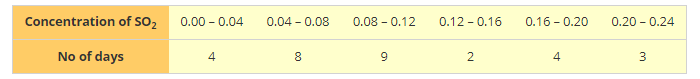# Given below is the frequency distribution table regarding the concentration of SO2 in the air in parts per million of a certain city for 30 days.

Question:

Given below is the frequency distribution table regarding the concentration of SO2 in the air in parts per million of a certain city for 30 days.Find the probability of the concentration of SO2 in the interval 0.12-0.16 on any of these days.

Solution:

Total no of days: 30

Probability of concentration of SO2 in interval 0.12 - 0.16

$=\frac{\text { Favorable out come }}{\text { Total out come }}$

= 2/30

= 1/15

= 0.06